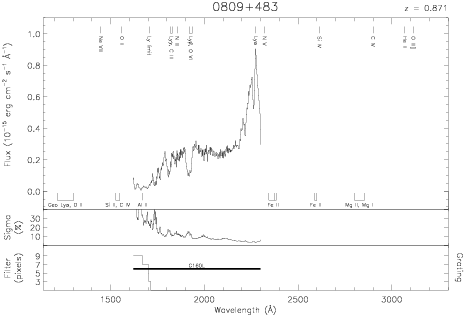Download dataset
TELESCOP= 'HST     '
INSTRUME= 'FOS     '
EQUINOX =               2000.0
DATE-OBS= '1991-12-04T02:52:06'
RA_PNT  =       123.4002458333
DEC_PNT =        48.2173583333
PA_PNT  =        70.0431530435
OBJECT  = '0809+483'
ROOTNM01= 'Y0PL0303T'
GRNDMD01= 'SPECTROSCOPY'
DETECT01= 'AMBER   '
APERID01= 'A-1     '
FGWAID01= 'L15     '
ROOTNM02= 'Y0ZW0202T'
DETECT02= 'AMBER   '
APERID02= 'B-3     '
FGWAID02= 'L15     '
ROOTNM03= 'Y0ZW0203T'